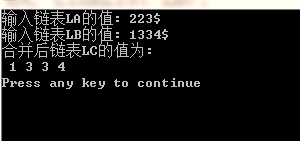# 代码如下

``````#include<stdio.h>
#include<stdlib.h>

typedef struct Node
{
char data;
struct Node * next;

int main()
{

InitList(&LA); //初始化单链表LA
printf("输入链表LA的值: ");
CreateFromTail(LA);

InitList(&LB); //初始化单链表LB
printf("输入链表LB的值: ");
CreateFromTail(LB);

printf("合并后链表LC的值为: ");
output(LC);

return 0;
}
{
(* L)->next = NULL;
}
{
Node * r, * s;
r = L;
int flag = 1;
char c;
while(flag)
{
c = getchar();
if(c != '\$')
{
s = (Node *)malloc(sizeof(Node));
s->data = c;
r->next = s;
r = s;
}
else
{
flag = 0;
r->next = NULL;
}
}
}
{
Node * pa, * pb, * r;
LC = LA; //将LC置为空表，且不额外分配结点空间
LC->next = NULL;
pa = LA->next; //让pa指向链表LA的第一个元素
pb = LB->next; //让pb指向链表LB的第一个元素
r = LC;

while(pa != NULL && pb != NULL)
{
if(pa->data <= pb->data)
{
r->next = pa;
r = pa;
pa = pa->next;
}
else
{
r->next = pb;
r = pb;
pb = pb->next;
}
}
if(pa)
r->next = pa;
else
r->next = pb;

free(LB);
return(LC);
}
{
while(p != NULL)
{
printf("%c ", p->data);
p = p->next;
}
printf("\n");
}
``````

# 运行结果2个回答

LC和LA都是指针，赋值之后，清空LC就等于清空LA了

``````     LinkList LC;
LC = LA; //将LC置为空表，且不额外分配结点空间
LC->next = NULL;
``````